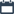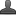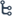## Tuesday, October 13, 2020

•October 13, 2020
••, ,
•No comments

## Andhra Pradesh Class-7 Mathematics Integers (chapter-1) Notes With Important Points

INTEGERS

Hi friends and my dear students! In this post, I have covered Andhra Pradesh class-7 Maths integers (chapter-1) important points After Reading Mathematics integers Notes With important points, Please do share it with your friends. You can Learn Maths for All Classes here.

Natural Numbers: The counting numbers 1,2,3,4,5,6.....are called  Natural numbers. It is represented by 'N'. N= (1, 2, 3, 4, 5, 6 ....}The smallest a natural number is 1 and the greatest natural number does not exist

Whole Numbers: Natural numbers including zero are called whole numbers. It is Represented by W. W= {0,1,2,3,4,5,6,... } The least whole number is 0 and the greatest whole number does not exist.

Integers: The set containing the positive numbers, the negative numbers together with zero is called the set of integers. Whole numbers including negative numbers are called Integers. It is represented by Z. The set of integers is represented by Z.

Z = {... - 4, -3, -2, -1, 0,1,2,3,4.....}

(or)

All the whole numbers together with negative of counting numbers form the set of all integers. Z or I= {...-5,-4,-3,-2,-1, 0, 1,2, 3..} is the set of all integers.

* Each time you add a positive integer, you move right on the number line.

* Each time you add a negative integer, you move left on the number line.

* -a' is called the additive inverse of a.

Note:

1{ 1.2,3,............} is called  Set of positive integers.

2. { -1,-2,-3,...............} is called  Set of negative integers. ,

3. Zero is considered neither positive nor negative.

4. In the number line all negative integers are on the left side of zero, all positive integers are on the right side of zero.

5. Set of positive integers and zero considered as Non – negative integers. {0,1,2,3,.......} is set of non – negative integers.

6. Set of negative integers and zero considered as Non - positive integers. (0,-1,-2,....}  is set of non - positive integers.

7. The differences between any two consecutive integers is 1.

8. The absolute value of an integer is the numerical value of the integer regardless of its sign.

Also Check

Introduction to Knowing Our Numbers Key Points

Trigonometry Do This & Try this solution

Introduction To Trigonometry

SSC (10th class) Trigonometry Exercise - 11.1 Solution

SSC(10th class) Trigonometry Exercise - 11.1 Solutions

9. Properties of Addition of Integers:

Let a € Z, b € Z, c € Z, then

i)Associative Law:

(a+ b) +c= a+ (b+c)

0+a=a+0 = a.  0' is the additive identity on integers

For each a € l there exist -a € l such that a + (-a) = (-a) +a=0.

10. i) The product of two integers with same sign is a positive integer.

ii) The product of two integers with unlike signs is a negative integer.

11. Properties of Multiplication of Integers:

Associative Law:

(a x b) x c =a x (b x c) for all integers a, b, c.

Distributive Law:

For all a,b,c € Z, we have a. (b+c)= a b+ a c and (a +b).c=a.c+b.c

Existence of Multiplicative Identity:

Clearly, 1 is an integer such that 1-a = a 1=a. Thus, 1 is the multiplicative identity. .

Multiplicative property of 0: For every integer a, we have a.0=0.a=0

On a number line when you add a positive integer you move right side on the number line and if a negative integer is added you move to the left side on the number line.

On the number line if you subtract a positive integer you move to the left side and if you subtract a negative integer you move to the right side.

Product of any two positive integers or any two negative integers is always a positive integer.

Product of a positive integer and a negative integer is always a negative integer two integers with opposite signs always give a negative product.

Product of even number of negative integers is always a positive integer.

Product of odd number of negative integers is always a negative integer